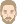## Highly-ventilated air gaps

ahayder
WUFI UserPosts: 2
Joined: Thu Apr 02, 2020 1:18 am -1100

### Highly-ventilated air gaps

Dear WUFI team,

In WUFI, adding an air-change source in the air layer does not automatically change the material properties of the air gap (the material properties remain of an unventilated air layer). Therefore, I want to create new materials to simulated highly-ventilated air gaps with different thicknesses (3 cm, 5 cm, and 10 cm). Could you please guide me to where I can find the basic properties of ventilated air gaps (such as Mu-value, moisture storage, permeability, etc.)? Thank you very much in advance!

ThomasPosts: 293
Joined: Sun Jun 19, 2005 10:33 pm -1100

### Re: Highly-ventilated air gaps

ahayder wrote:
Thu Apr 02, 2020 1:41 am -1100
I want to create new materials to simulated highly-ventilated air gaps with different thicknesses (3 cm, 5 cm, and 10 cm). Could you please guide me to where I can find the basic properties of ventilated air gaps (such as Mu-value, moisture storage, permeability, etc.)?
Dear ahayder,
I assume that by "highly ventilated air gaps" you mean air gaps in which air flows with non-negligible speed, the flow being forced by external conditions (such as pressure differences).

The bulk properties of the air (density, heat capacity, moisture storage function, etc.) will be more or less unaffected by the fact that the air is flowing. Pressure changes occurring along the flow path will affect these properties slightly, but in the context of building physics these effects will usually be negligible.

But the transport properties (describing heat flow and vapor diffusion flow) will be affected substantially. Currently, WUFI's air layers model the effect of the air flow as described by tables in the building physics text book of Gösele and Schüle. We are planning a revision of the air layers used by WUFI, according to the following considerations.

The aim is to describe the heat flow through a plane air layer between two bounding surfaces. Part of the heat flow is due to the exchange of thermal radiation between the two bounding surfaces (including mutiple reflection), this is easily calculated by basic physics.

The heat flow due to conduction and convection in the air is more complex. It can be described by similarity theory, that is, by using appropriate dimensionless numbers. The heat flow across the air layer is described by the Nusselt number of the system. On the other hand, the conditions giving rise to the flow (in our case free convection, driven by temperature differences) can be described by the Prandtl number and the Grashof number of the system. Similarity theory shows that (under certain circumstances) there is a universal relationship giving the Nusselt number in terms of the other two numbers: Nu = f(Pr, Gr).

For given conditions, in particular the given bounding surface temperatures, the corresponding Prandtl and Grashof numbers can be calculated, this allows the Nusselt number to be calculated, and for WUFI we then simply compute the effective lambda which - for a given air layer thickness - gives the correct Nusselt number for this air layer. This procedure is described, for example, in the standard document "DIN EN 673 - Glass in building - Determination of thermal transmittance (U-value) - Calculation method".

As mentioned above, this approach applies to the case of "free" or "natural" convection where the air flow is caused by buoyancy effects. What you wish to consider, however, is the case of "forced" convection. In this case the Nusselt number of the system is a function of the Prandtl number and the Reynolds number of the system. You will have to find out what this function (a.k.a "correlation") is for plane air layers. Sorry, I don't have formulas at hand, but I'm confident that there exists literature about such a "simple" system. A good starting point may be

Bergman, Lavine, Incropera, Dewitt: Fundamentals of Heat and Mass Transfer

but I can't look it up right now because it's in my office and I'm working from home.

So far, this was about thermal transport across an air flow. We still need to consider diffusion flow. The formula used for determining the effective mu-values of WUFI's air layers from their effective thermal conductivities is described in WUFI's help file. This formula is in agreement with the experimental results found by

H K Wee: Heat and Mass Transfer in Confined Spaces
PhD thesis, Uni Canterbury, 1986

where free convection in an air space is investigated. I don't know to which extent the results also apply to forced convection.

Regards,
Thomas

ahayder
WUFI UserPosts: 2
Joined: Thu Apr 02, 2020 1:18 am -1100

### Re: Highly-ventilated air gaps

Thank you very much for the comprehensive reply!